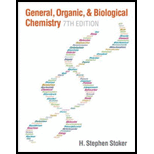# The total number of atoms present in a formula unit of Al 2 (SO 4 ) 3 is a. 15 b. 16 c. 17 d. no correct response### General, Organic, and Biological C...

7th Edition
H. Stephen Stoker
Publisher: Cengage Learning
ISBN: 9781285853918

#### Solutions

Chapter
Section### General, Organic, and Biological C...

7th Edition
H. Stephen Stoker
Publisher: Cengage Learning
ISBN: 9781285853918
Chapter 1.10, Problem 6QQ
Textbook Problem
1 views

## The total number of atoms present in a formula unit of Al2(SO4)3 is a. 15 b. 16 c. 17 d. no correct response

Interpretation Introduction

Interpretation:

Total number of atoms that is present in a formula unit of Al2(SO4)3 has to be chosen from the given options.

Concept Introduction:

The composition of a chemical compound can be presented in a very precise was with the use of chemical formula.  Chemical formula uses chemical symbols of the elements that is present in the compound and numerical subscripts that represent how many number of atoms of each element is present in the compound.

### Explanation of Solution

Reason for correct option:

Given formula unit is Al2(SO4)3.  The total number of atoms can be found by summing up the subscript as shown below,

TotalNo.ofatomsinAl2(SO4)3 = 2+(1x3)+(4x3)

### Still sussing out bartleby?

Check out a sample textbook solution.

See a sample solution

#### The Solution to Your Study Problems

Bartleby provides explanations to thousands of textbook problems written by our experts, many with advanced degrees!

Get Started

Find more solutions based on key concepts
Both carbohydrates and protein have 4 calories per gram. T F

Nutrition: Concepts and Controversies - Standalone book (MindTap Course List)

What major conclusions were drawn from the Rutherford scattering experiment?

Introductory Chemistry: An Active Learning Approach

How are extremophiles thought to produce energy?

Oceanography: An Invitation To Marine Science, Loose-leaf Versin

The switch in Figure P27.51a closes when Vc23Vand opens when Vc13V. The ideal voltmeter reads a potential diffe...

Physics for Scientists and Engineers, Technology Update (No access codes included)